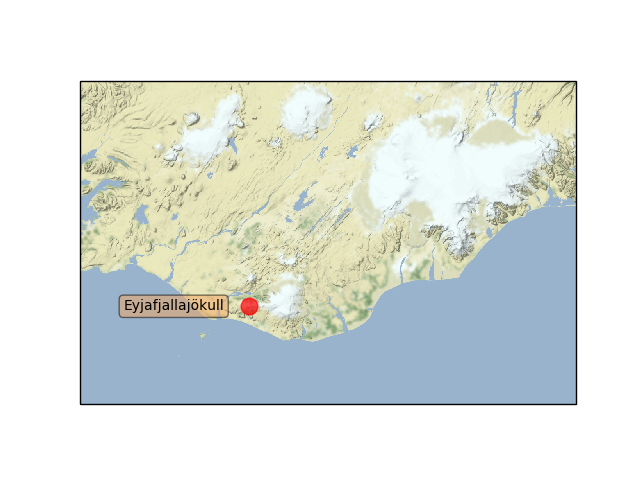# Map tile acquisition¶

Demonstrates cartopy’s ability to draw map tiles which are downloaded on demand from the Stamen tile server. Internally these tiles are then combined into a single image and displayed in the cartopy GeoAxes.

## Grabbing the map tiles¶```import matplotlib.pyplot as plt
from matplotlib.transforms import offset_copy

import cartopy.crs as ccrs
import cartopy.io.img_tiles as cimgt

def main():
# Create a Stamen Terrain instance.
stamen_terrain = cimgt.StamenTerrain()

fig = plt.figure()

# Create a GeoAxes in the tile's projection.
ax = fig.add_subplot(1, 1, 1, projection=stamen_terrain.crs)

# Limit the extent of the map to a small longitude/latitude range.
ax.set_extent([-22, -15, 63, 65], crs=ccrs.Geodetic())

# Add the Stamen data at zoom level 8.

# Add a marker for the Eyjafjallajökull volcano.
ax.plot(-19.613333, 63.62, marker='o', color='red', markersize=12,
alpha=0.7, transform=ccrs.Geodetic())

# Use the cartopy interface to create a matplotlib transform object
# for the Geodetic coordinate system. We will use this along with
# matplotlib's offset_copy function to define a coordinate system which
# translates the text by 25 pixels to the left.
geodetic_transform = ccrs.Geodetic()._as_mpl_transform(ax)
text_transform = offset_copy(geodetic_transform, units='dots', x=-25)

# Add text 25 pixels to the left of the volcano.
ax.text(-19.613333, 63.62, u'Eyjafjallajökull',
verticalalignment='center', horizontalalignment='right',
transform=text_transform,
bbox=dict(facecolor='sandybrown', alpha=0.5, boxstyle='round'))
plt.show()

if __name__ == '__main__':
main()
```

Total running time of the script: ( 0 minutes 0.045 seconds)

Generated by Sphinx-Gallery